Math Calculators
Long Division Calculator

# Long Division Calculator

Long division calculator performs long division with remainders. Demonstrates solution with steps, and answer as quotient and remainder and as mixed number.

17÷3 = 5 R 2 = 5 2/3

There was an error with your calculation.This long division calculator performs long division with remainders. It divides one of the given numbers (dividend) with another number (divisor) and presents the answer in terms of a whole number (quotient) and a remainder. The answer is also provided in a mixed number form. The resulting mixed number is simplified if it is possible.

## Directions for use

• To use the division with the remainders calculator, enter Dividend and Divisor in the corresponding fields and press “Calculate.” The calculator will return the long division result as a quotient with remainder, mixed number, and mixed number in its simplest form. The solution algorithm will also be demonstrated.
• Note that only positive integers (whole numbers) are accepted as inputs. And the dividend should be larger than the divisor.

## Calculation algorithm

You can perform long division with remainders or long division with decimals. Here we focus on the former – division with remainders.

### Definitions

• Dividend is the number you are dividing, the larger of the two numbers.
• Divisor is the number you are dividing with, the smaller of the two numbers.
• Quotient is the whole number part of the answer.
• Remainder is the left-over number.

For example, 168 / 15 = 11 R3, where 168 is the dividend, 15 is the divisor, 11 is the quotient, and 3 is the remainder.

## Long division with remainders algorithm

The steps for performing the division are demonstrated in the text below. Let’s consider the division steps, as applied to the above example: 168 / 15.

### Step 1

• Write down the divisor and the dividend next to each other, starting with the divisor.
• Separate the divisor and the dividend by a vertical line.
• Draw a horizontal line over the dividend, to separate it from the quotient.

The combination of the horizontal and vertical lines is usually referred to as the division bracket or the division symbol. Note that the division bracket is included in the calculator interface for convenience.### Step 2

• Divide the first digit of the dividend by the divisor. In this case, divide 1 by 15. 1 divided by 15 equals 0 with a remainder of 1.
• Write the whole number part of the division above the horizontal line. In this example, you will write 0. The numbers above the horizontal line will form the quotient part of the answer.
• Multiply the whole number part of the division (0 in our case) by the divisor (15) and write the result (0) under the first digit of the dividend. Draw a horizontal line under this number to finish step 2.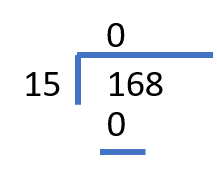### Step 3

• Subtract the whole number part of the division in step 2 from the first digit of the dividend: 1 – 0 = 1. Write the answer (1) under the horizontal line.
• Bring down the second digit of the dividend (6), and write it next to that answer. In our example, we’ll have 16.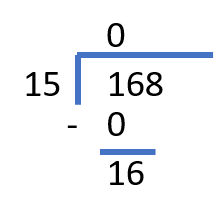### Step 4

Repeat step 2 for the new number: 16.

• Divide the new number (16) by the divisor (15). 16 divided by 15 equals 1 with a remainder of 1.
• Write the whole number part of the division, 1, above the horizontal line.
• Multiply the whole number part of the division (1) by the divisor (15) and write the result under 16. 1 × 15 = 15. Draw a horizontal line under this number to finish step 4.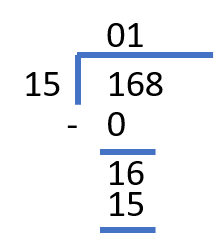### Step 5

Repeat step 3 with the new numbers.

• Subtract the whole number part of the division in step 4 from the new number: 16 – 15 = 1. Write the answer (1) under the horizontal line.
• Bring down the third digit of the dividend, and write it next to that answer. In our example, the resulting new number will be 18.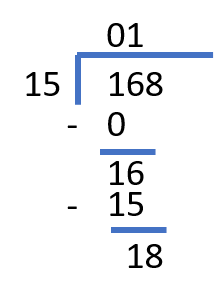### Step 6

Repeat step 2 for the new number: 18.

• 18 divided by 15 equals 1 with a remainder of 3.
• Write 1 above the horizontal line.
• 1 × 15 = 15. Write 15 under 18.
• Draw a horizontal line to finish step 6.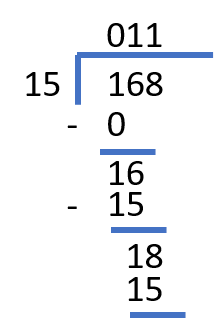### Step 7

Start repeating step 3 with the new numbers.

18 – 15 = 3

You do not have any new digits, and you can’t bring down any new number. 3 is less than 15; therefore, the division is finished. The final number under the horizontal line is the remainder of the division. The number above the division bracket is the quotient part of the answer.

168 / 15 = 11 R3

You can also write the answer in a mixed number form:

168 / 15 = 11 3/15

Or, in a simplified form:

168 / 15 = 11 1/5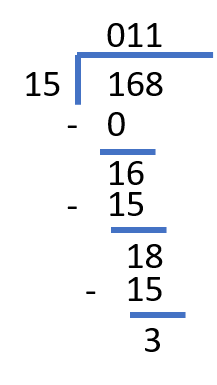## Calculation examples

### Example 1

Patrick was given $150 for his birthday. He likes toy railways and wants to update his collection of trains. Each train costs$11. How many trains can Patrick buy? How much money will he have left?

Solution

To find the solution to this problem, we need to perform long division with remainders. The quotient part of the answer will represent the number of trains Patrick can buy, and the remainder will represent the amount of money he will have left.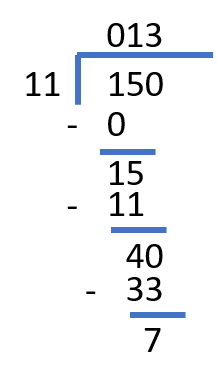150 / 11 = 13 R7.

Patrick can buy 13 trains. He will have \$7 left.

### Example 2

Jane is filling up treat bags to bring to the class on her birthday. She has two big packages of Haribo bears, each with 65 pieces inside. Jane wants to put 8 bears in each treat bag. How many full treat bags can she make? If any bears are left over, Jane is allowed to eat them. Will there be anything for Jane to eat, and if yes, how many bears can she have?

Solution

To find the solution to the problem, we should perform long division with remainders. The quotient part of the answer will represent the amount of full treat bags. The remainder of the answer will represent the number of gummy bears Jane can eat.

First, let’s calculate the dividend for the long division. There are 2 packages with 65 gummy bears each; therefore, there are 2 × 65 = 130 bears.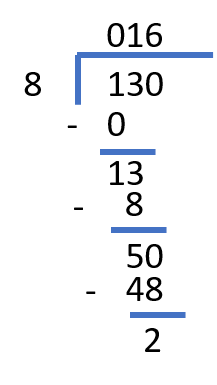130 / 8 = 16 R2.